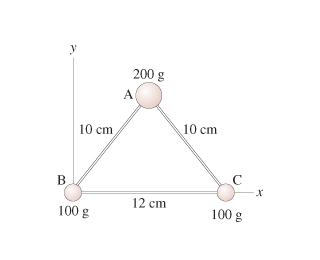# Problem: Find the coordinates of the center of mass. Find the x-coordinate.Express your answer to two significant figures and include the appropriate units.

🤓 Based on our data, we think this question is relevant for Professor Tolich's class at UW-SEATTLE.

###### FREE Expert Solution

Center of mass about an axis is given by:

$\overline{)\begin{array}{rcl}\mathbf{\sum }_{\mathbf{n}\mathbf{=}\mathbf{1}}^{\mathbf{n}\mathbf{=}\mathbf{i}}{\mathbf{m}}_{\mathbf{i}}{\mathbf{x}}_{\mathbf{i}}& {\mathbf{=}}& \mathbf{\left(}\mathbf{\sum }_{\mathbf{n}\mathbf{=}\mathbf{1}}^{\mathbf{n}\mathbf{=}\mathbf{i}}{\mathbf{m}}_{\mathbf{i}}\mathbf{\right)}\overline{\mathbf{x}}\end{array}}$

One of the 100 g masses is located at x = 0 cm while the other is located at x = 12 cm. The 200 g mass is located in an equilateral triangle so that x = 6 cm.###### Problem DetailsFind the coordinates of the center of mass. Find the x-coordinate.

Express your answer to two significant figures and include the appropriate units.